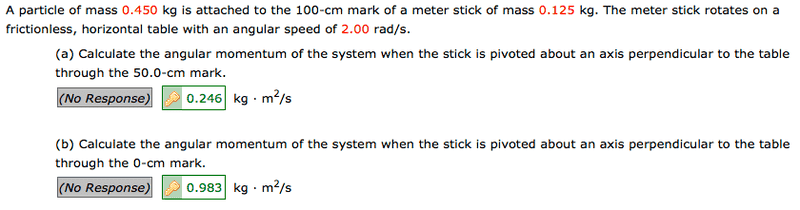# Calculate angular momentum? I almost got the right answer but it's wrong Help!

Calculate angular momentum? I almost got the right answer but it's wrong!! Help!This is what I did for part a...

1. What is given

Particle
mp = 0.450 kg
ω = 2.00 rad/s
r = 1 m

Stick
ms = 0.125 kg
ω = 2.00 rad/s
r = 0.5 m

2. Angular momentum is conserved (Linitial = Lfinal) due to a lack of friction

0 = Lfinal

L = Iω
L = (mr2
L = (0.450 + 0.125)(0.52)(2)
L = (0.575)(0.25)(2)
L = 0.2875

Help!!!!

## Answers and Replies

TSny
Homework Helper
Gold Member

2. Angular momentum is conserved (Linitial = Lfinal) due to a lack of friction

0 = Lfinal
?? This problem does not involve conservation of angular momentum.

L = Iω
L = (mr2

You need to find the moment of inertia of the stick either by calculation using calculus or by consulting a table.